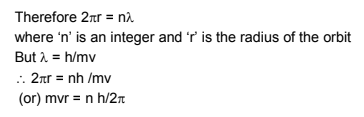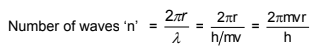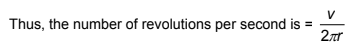# Derivation of Angular Momentum from de Broglie Equation

According to Bohr’s model, the electron revolves around the nucleus in circular orbits.

According to de Broglie concept, the electron is not only a particle but has a wave character also.

If the wave is completely in phase, the circumference of the orbit must be equal to an integral multiple of wave length.which is Bohr’s postulate of angular momentum, where ‘n’ is the principal quantum number.

“Thus, the number of waves an electron makes in a particular Bohr orbit in one complete revolution is equal to the principal quantum number of the orbit”.

Alternativelywhere v and r are the velocity of electron and radius of that particular Bohr orbit in which number of waves are to be calculated, respectively.

The electron is revolving around the nucleus in a circular orbit. How many revolutions it can make in one second Let the velocity of electron be v m/sec. The distance it has to travel for one revolution 2r, (i.e., the circumference of the circle).Common unit of energy is electron volt which is amount of energy given when an electron is accelerated by a potential of exactly 1 volt. This energy equals the product of voltage and charge. Since in SI units coulombs  volts = Joules, 1 eV numerically equals the electronic charge except that joule replaces coulombs.

Post By : Ramashankar Rajppot 16 Jan, 2020 2315 views Chemistry